﻿ 20-sim webhelp > Modeling Tutorial > Bond Graphs > From Bond Graph to Block Diagram

# From Bond Graph to Block Diagram

With a causal bond graph model, equivalent block diagram models can easily be derived. To create a block diagram, the following steps have to be performed.

 • Change bonds by equivalent bilateral signals.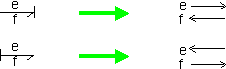• Replace elements by corresponding block diagram symbols. Use the correct effort and flow description which can be found in the element tables. As an example both descriptions for the R-element are given.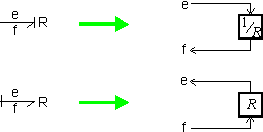• Replace the junctions by signal summation points and signal splitters. If correct, the bonds have already be replaced by effort and flow signals. Out of these signals and the junction description you can derive the effort and flow equations for the junction. As an example the conversion for a 1 junction is shown.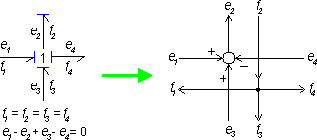## Example

As an example in the figure below a causal bond graph model is shown.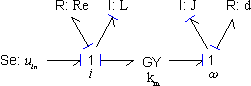Using the given set of rules and the element descriptions an equivalent block diagram models is found, which is shown below.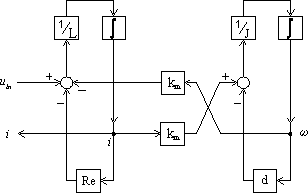The resulting block diagram model can be simplified by combining blocks and elimination of loops. Out of the block diagram, easily a set of dynamic equations can be deduced.